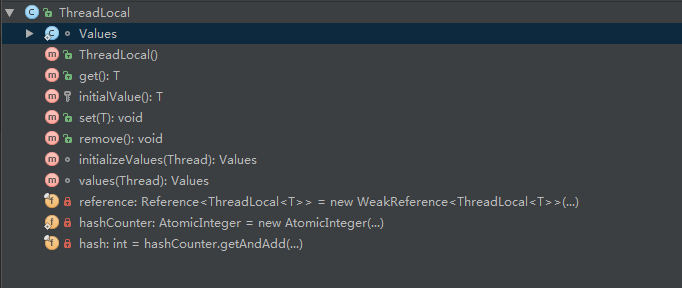JDK 1.6文档是这样定义的:

Map存储的时候，线程并发访问，数据都不同

    private static Map<Integer, Integer> map = new HashMap<>();
private static void test2() {
for (int i = 0; i < 4; i++) {
@Override
public void run() {
int n = 0;

if (map.get(0) != null) {
n = map.get(0);
}

for (int j = 0; j < 5; j++) {
n++;
}

map.put(0, n);

}
}
}

ThreadLocal存储数据，每个数据都是独立的，线程之间没有任何干扰

 private static ThreadLocal<Integer> threadLocal = new ThreadLocal<>();

private static void test() {
for (int i = 0; i < 4; i++) {
@Override
public void run() {
int n = 0;

}

for (int j = 0; j < 5; j++) {
n++;
}

}
}
}/** Weak reference to this thread local instance. */
private final Reference<ThreadLocal<T>> reference= new WeakReference<ThreadLocal<T>>(this);

private static AtomicInteger hashCounter = new AtomicInteger(0);
private final int hash = hashCounter.getAndAdd(0x61c88647 * 2);

hashCounter是线程安全的加减操作，getAndSet(int newValue)取当前的值，并设置新的值；而0x61c88647 * 2作用是:Value存在数据的主要存储数组table上,而table被设计为下标为0,2,4…2n的位置存放key，而1,3,5…(2n +1 )的位置存放value,0x61c88647 * 2保证了其二进制中最低位为0，也就是在计算key的下标时，一定是偶数位

- public T get()：返回此线程局部变量的当前线程副本中的值

    public T get() {
// Optimized for the fast path.
// 获取当前线程
// 获取当前线程的Value实例
if (values != null) {
Object[] table = values.table;
// 如果键值的key的索引为index，则所对应到的value索引为index+1.
int index = hash & values.mask;
if (this.reference == table[index]) {
return (T) table[index + 1];
}
} else {
// 如果当前Value实例为空，则创建一个Value实例
}

return (T) values.getAfterMiss(this);
}

initializeValues(currentThread)主要是直接new出一个新的Values对象

   Values initializeValues(Thread current) {
return current.localValues = new Values();
}
• protected T initialValue() 返回此线程局部变量的当前线程的“初始值”。
  protected T initialValue() {
return null;
}

• public void set(T value) 将此线程局部变量的当前线程副本中的值设置为指定值.
    public void set(T value) {
// 获取当前线程
// 获取当前线程的Value实例
if (values == null) {
}
// 将数据设置到Value中
values.put(this, value);
}
• public void remove() 移除此线程局部变量当前线程的值。

Values内部存储数据，请看Android 线程本地变量<二> ThreadLocal Values源码解析`博客©️2019 CSDN 皮肤主题: 大白 设计师: CSDN官方博客